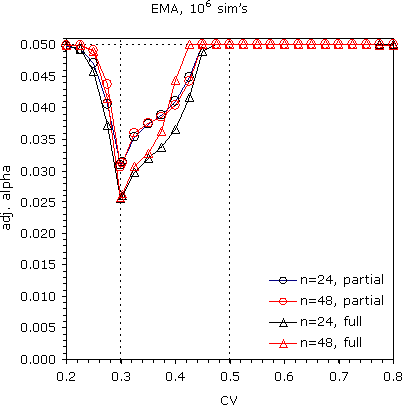## If it is real: results [RSABE / ABEL]

Hi Martin!

» IMHO: yes as this is a constant and should not dependent on the number of simulation runs.

Oh yes, sure. Sorry for my stupidity.

» Ps.: ... and yes its the same guy who is famous for publicly calling some approaches “bullshit”I always thought he should work on his social skills.

Here the results of my sim’s for target α 0.05. Partial and full replicate designs, n=24/48.The FDA’s method would lead to a large α-inflation (as presented by Detlew above) for CVWR ≤30%. This could be corrected by adjusting α. However, since scaling is only allowed for CVWR >30% nothing has to be done. Like the conventional TOST the test gets more con­ser­vative with increasing CV (and n).The EMA’s method is another cup of tea. α-inflation is seen up to 45%! The test is closer to the nominal level than the FDA’s.
Interesting the behavior of the partial replicate with n=24.Code (10–25 iterations to reach convergence, runtime on my machine 15–45 seconds depending on design, n, CV):
require(PowerTOST) reg   <- "EMA"   # "EMA" for ABEL or "FDA" for scABE CV    <- 0.300   # intra-subject CV of reference n     <- 24      # total sample size                  # in case of imbalanced sequences use a vector:                  # e.g., c(n1,n2,n3) des   <- "2x3x3" # partial: "2x3x3" full: "2x2x4"                  # for others see known.designs() print <- TRUE    # intermediate results if (reg == "EMA") { # scABEL   ifelse (CV <= 0.5, GMR <- exp(0.7601283*CV2se(CV)),                      GMR <- exp(0.7601283*CV2se(0.5)))   if (CV <= 0.3) GMR <- 1.25   } else {          # RSABE   ifelse (CV > 0.3, GMR <- exp(0.8925742*CV2se(CV)),                     GMR <- 1.25) } nsims <- 1e6 prec  <- 1e-8    # precision of bisection algo x     <- 0.05    # target alpha nom   <- c(0.001, x)                # from conservative to target alpha lower <- min(nom); upper <- max(nom) delta <- upper - lower              # interval ptm   <- proc.time()                # start timer iter  <- 0 while (abs(delta) > prec) {         # until precision reached   iter  <- iter + 1   x.new <- (lower+upper)/2          # bisection   if (reg == "EMA") {     pBE <- power.scABEL(alpha=x.new, theta0=GMR, CV=CV,                         n=n, design=des, nsims=nsims)     } else {     pBE <- power.RSABE(alpha=x.new, theta0=GMR, CV=CV,                        n=n, design=des, nsims=nsims)   }   if (print) {                      # show progress     if (iter == 1) cat(" i  adj. alpha       pBE\n")     cat(format(iter, digits=2, width=2),         format(x.new, digits=6, width=11, nsmall=7),         format(pBE, digits=6, width=9, nsmall=6), "\n")     if (.Platform\$OS.type == "windows") flush.console()   }   if (abs(pBE - x) <= prec) break   # precision reached   if (pBE > x) upper <- x.new       # move upper limit downwards   if (pBE < x) lower <- x.new       # move lower limit upwards   delta <- upper - lower            # new interval } if (print) cat("run-time:", round((proc.time()-ptm), 1),                "seconds  iterations:", iter, "\n") cat("regulator:", reg, " CV:", CV, " n:", n, " design:", des,     "\ntarget alpha:", x, " adjusted alpha:", x.new,     " pBE:", pBE, "(empiric alpha)\n")

Dif-tor heh smusma 🖖
Helmut SchützThe quality of responses received is directly proportional to the quality of the question asked. 🚮
Science QuotesIng. Helmut Schütz